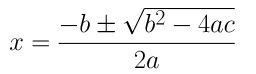Guru

# Find the roots of the following equations: (i) x-1/x = 3, x ≠ 0 Q.3(1)

• 0

How i find the root of (i) x-1/x = 3, x ≠ 0 , because it very important question.

Share

1. x-1/x = 3

⇒ x2 – 3x -1 = 0

On comparing the given equation with ax2 + bx + c = 0, we get

a = 1, b = -3 and c = -1

By using quadratic formula, we get,⇒ x = 3±√9+4/2

⇒ x = 3±√13/2

∴ x = 3+√13/2 or x = 3-√13/2

• 0